Scaling behaviour for the water transport in nanoconfined geometries

Abstract

The transport of water in nanoconfined geometries is different from bulk phase and has tremendous implications in nanotechnology and biotechnology. Here molecular dynamics is used to compute the self-diffusion coefficient D of water within nanopores, around nanoparticles, carbon nanotubes and proteins. For almost 60 different cases, D is found to scale linearly with the sole parameter θ as D(θ)=DB[1+(DC/DB−1)θ], with DB and DC the bulk and totally confined diffusion of water, respectively. The parameter θ is primarily influenced by geometry and represents the ratio between the confined and total water volumes. The D(θ) relationship is interpreted within the thermodynamics of supercooled water. As an example, such relationship is shown to accurately predict the relaxometric response of contrast agents for magnetic resonance imaging. The D(θ) relationship can help in interpreting the transport of water molecules under nanoconfined conditions and tailoring nanostructures with precise modulation of water mobility.

Introduction

Despite its fundamental importance in science and technology, the physical and transport properties of water are far from being completely understood1. The self-diffusion of water molecules D in proximity of solid surfaces, at the interface between immiscible liquids, and in confined geometries, such as nanopores and nanotubes, is a very different process as compared to the bulk phase2,3,4. The thermal agitation of the water molecules in the bulk liquid is only dictated by the local temperature and pressure conditions, and molecular diffusion follows the Einstein relation5. Differently, under confined conditions, the mobility of water molecules is perturbed by the presence of additional interaction forces arising at the water/solid interfaces, mainly van der Waals and Coulomb interactions. These additional forces usually reduce the local molecular diffusion6,7. Even if considerable work has been done in recent years, both experimentally and theoretically, to understand and characterize the perturbed behaviour of the water molecules in confined geometries, there is still no complete comprehension of the process and often the published results are contradictory8.

Controlling the mobility of water molecules is of relevance to several scientific disciplines and has implications in multiple technological applications. For instance, water adsorption/desorption in nanoporous materials, such as zeolites, has potential in long-term thermal storage and energy engineering9,10; filters with nanopores and nanochannels are increasingly explored for their large surface area and higher efficiency11,12; in heat transfer problems, nanofluids are under investigation because of their peculiar thermal properties13,14; in micro/nanotechnology processes, controlling the deposition and surface diffusion of water molecules is critical for precise manufacturing15,16; in biology, the mechanisms regulating the transport of single water molecules through cell membrane channels (aquaporins) and the multi-scale water compartmentalization in tissues are still elusive17,18,19,20. Also, proteins tend to modify their structure and function according to the surrounding aqueous environment21,22.

Certainly, nanomedicine is one of the fields where several exciting discoveries and technological applications can be directly related to the anomalous behaviour of water in confined geometries. A few examples are the enhancement in longitudinal relaxivity associated with the entrapment of Gd3+-ion complexes in mesoporous structures23,24; the dynamics of water molecules in nanotubes and nanochannels for controlled drug delivery25,26; and the design of hydrogel-based nano/microparticles27,28. In particular, the dynamics of water molecules is essential in magnetic resonance imaging (MRI), in that contrast enhancement is influenced by the local diffusion of water molecules29,30. It is known that for paramagnetic metal complexes, such as Gd3+ ions, the Solomon–Bloembergen–Morgan theory31 would predict a change in longitudinal relaxivity r1 of the complex following a variation in the relative translational diffusion time (τD) of the water molecules surrounding the complex, and in the residence lifetime (τM) of the water molecules bound to the complex. Similarly, for magnetic nanoparticles (NPs), such as the iron oxide NPs, an increase in τD (that is, decrease in D) would enhance the transversal relaxivity r2 (ref. 32). Hence, the modulation and precise control of the diffusion of the water molecules in the vicinity of an MRI contrast agent plays an important role in imaging performance. This concept has been already successfully proved by experiments23, but a clear rationale (and a computationally efficient tool) for optimally designing such agents is still missing.

In this work, the self-diffusion coefficient D of water molecules is investigated through molecular dynamics (MD) simulations under five different isothermal configurations, namely, within silica (SiO2) nanopores, around spherical hydroxylated NPs, within SiO2 nanopores filled by NPs, around single-wall carbon nanotubes (CNTs) and proteins. The coefficient D has been estimated for almost 60 cases by varying the size of the NPs and nanopores, the electrostatic surface charges and level of hydrophobicity, as well as the type of protein. The self-diffusion coefficient D for all different configurations has been found to scale with a single non-dimensional parameter θ, incorporating both geometrical and physicochemical information, following the relationship D(θ)=DB[1+(DC/DB−1)θ]. The D(θ) scaling is modulated by the coefficients DB and DC, which represent the bulk and totally confined diffusion of water, respectively. This D(θ) law has been applied to estimate the enhancement in MRI contrast in magnetic nanoconstructs obtained by geometrically confining super-paramagnetic iron oxide NPs (SPIOs) into silicon mesoporous matrices. It has been confirmed that the transversal relaxivity of SPIOs can be significantly augmented by modulating the diffusion of water molecules. This law would help in explaining and rationalizing previous experimental evidences23, and represent a ready-to-use tool for the rational design of nanoconstructs based on the nanoscale confinement of water molecules.

Results

Computing the diffusion of nanoconfined water molecules

MD simulations were used to compute the self-diffusion coefficient D of water molecules confined under different configurations. These are shown in Fig. 1 and include the case of water molecules (blue dots) moving (a) around spherical hydroxylated nanoparticles (NPs) (grey dots); (b) within a hydrated nanopore (grey dots); (c) around hydroxylated NPs (red dots) adsorbed on the surface of a hydrated nanopore (grey dots); (d) around and within single-walled carbon nanotubes (CNTs); (e,f) around proteins. The NPs are made out of magnetite (Fe3O4) crystals (red and cyan dots), with OH functional groups on their surface, or SiO2 crystals (grey dots), with silanol SiOH functional groups on the surface. The nanopores are made out of SiO2 only.

To investigate the influence of geometry and material properties, the self-diffusion coefficient D of the water molecules was computed for 58 different cases. In particular, these cases were different in terms of NP diameter, being 1.3, 2.0 or 5.2 nm; nanopore diameter, with values 2.0, 4.1, 8.1 and 11.0 nm; number of NPs adsorbed on the nanopore wall, varying from 0 to 66 NPs per pore; CNT armchair chirality, namely, (5,5), (10,10), (20,20) and (30,30); and type of proteins, including molecules with a spherical (for example, ubiquitin) and elongated (for example, Ca2+-ATPase) shapes; of small (for example, 562 atoms of B1-immunoglobulin-binding domain) and large (for example, 9667 atoms of Ca2+-ATPase) sizes; and exhibiting a catalytic (for example, glucokinase), hormonal (for example, leptin) and transport (for example, myoglobin) function. As per the material properties, two types of interactions were considered in the MD simulations: bonded interactions and non-bonded interactions between the water molecules and the solid surfaces, described via van der Waals and Coulomb potentials. In the SiO2 structures and spherical NPs, the bonded interactions are modelled by means of harmonic terms. For the NPs, the strength ε of the Lennard–Jones potential was varied from 2.49 to 24.94 kJ mol−1 and the partial electrostatic charges of atoms were set to either the nominal value or zero for NPs and nanopores. For simulations with CNTs, the non-bonded interactions between CNTs and water molecules were modelled by the Lennard–Jones potential, with neutral carbon atoms and σCC=0.36 nm, εCC=0.29 kJ mol−1 (ref. 33). Finally for the proteins, all bonded and non-bonded interactions were modelled using the GROMOS96 43a2 force field34, which has been widely used for studying similar applications35,36. Finally, various hydration levels were considered providing an overall water density ranging from 715 to 941 kg m−3. Note that for these density values, there is no heterogenous wetting and consequently no anomalous behaviour related to low water-filling regimes3,7.

All computed values of the self-diffusion coefficient D are reported in the Supplementary Tables 1–11 and Supplementary Note 1. In general, it is observed that the coefficient D decreases compared with the bulk value, 2.60 × 10−9 m2 s−1 at 300 K (ref. 37), as the ratio between the total area of the solid–liquid interface and the total volume occupied by the water (Vw) increases. More specifically, for a SiO2 nanopore, D is reduced from 2.50±0.09 × 10−9 m2 s−1 to 0.82±0.22 × 10−9 m2 s−1 as the pore diameter decreases from 11.0 to 2.0 nm. Moreover, D is inversely proportional to the NP concentration and diameter. For a 5.2-nm SiO2 NP, D decreases from the almost unconfined value to 2.12±0.04 × 10−9 m2 s−1 following a 36% increase in NP concentration. Indeed, the increase in NP concentration is associated with a decrease in separation distance between adjacent NPs and, consequently, a decrease in the volume available to water molecules. Consistently, D reduces as the concentration of Fe3O4 NP increases within a nanopore: D is 2.20±0.10 × 10−9 m2 s−1 in an 8.1-nm SiO2 nanopore; however, if 2 Fe3O4 NPs, of 2.0 nm in diameter, are adsorbed on the nanopore surface, D decreases to 2.07±0.14 × 10−9 m2 s−1 and it drops to 0.44±0.05 × 10−9 m2 s−1 (~80% decrease), if 16 Fe3O4 NPs, of 2.0 nm in diameter, are added in the nanopore. In addition, for 16 Fe3O4 NPs adsorbed on the wall of an 8.1-nm nanopore, D is 1.46±0.09 × 10−9 m2 s−1 for 1.3 nm NPs and becomes 0.44±0.05 × 10−9 m2 s−1 (~70% decrease) for 2.0 nm NPs. A similar trend is observed for the CNTs and proteins by reducing the size of the water box. More specifically, around the (5,5) chirality CNT with a length of 5 nm, the water diffusivity D decreases from the bulk value (box of 316 nm3) to 1.22±0.12 × 10−9 m2 s−1 (box of 21 nm3). Similarly, around the B1-immunoglobulin-binding domain, the diffusivity D decreases from 2.41±0.04 × 10−9 m2 s−1 (box of 348 nm3) to 0.87±0.10 × 10−9 m2 s−1 (box of 23 nm3). As expected, the above data qualitatively demonstrate that the self-diffusion coefficient D of water is strongly correlated to the ratio between the interface surface and the total water volume: the larger this ratio the smaller the water mobility. However, the possible contribution of other parameters should also be assessed.

To this end, sensitivity analyses were performed to elucidate the effect of the Lennard–Jones potential strength ε and Coulomb interactions on D. Larger values of the parameter ε are associated with lower mobility of the water molecules. As an example, let us consider the case of eight Fe3O4 NPs (2.0 nm diameter) adsorbed on the walls of an 8.1-nm diameter SiO2 nanopore. A one order of magnitude decrease of ε of Fe3O4 atoms only carries a 10% increase of D: for ε=24.94 kJ mol−1 D is equal to 1.33±0.13 × 10−9 m2 s−1, whereas D increases to 1.47±0.11 × 10−9 m2 s−1 for ε=2.49 kJ mol−1. Moreover, D increases as the surface electrostatic charges decrease. For neutral Fe3O4 NPs, D grows to 1.64±0.04 × 10−9 m2 s−1 and, if both the NPs and nanopore wall are electrically neutral, D takes the value of 1.69±0.20 × 10−9 m2 s−1 (~30% increase as compared with the example above). Although the water molecule confinement is affected by the strength of the interaction potentials (van der Waals and Coulomb), geometrical parameters show a greater influence on the coefficient D. The reason is that all considered surfaces have effective wall potentials that are strong enough to induce a significant reduction of the water mobility in a region close to the wall. On the other hand, as clarified below, the volume of the low mobility region only slightly depends on the wall potential strength, namely, the minimum of the potential well generated by the wall.

Finally, the self-diffusion coefficient D did not change significantly with the level of hydration in the considered range, in accordance with previous studies7. Considering again the representative case of eight Fe3O4 NPs, of 2.0 nm in diameter, adsorbed on the wall of an 8.1-nm SiO2 nanopore, D ranges from 1.30±0.11 × 10−9 to 1.40±0.07 × 10−9 m2 s−1 (<10% variation) as the water density increases from 700 to 930 kg m−3.

Characteristic length of confinement

In the bulk fluid, the water molecules fluctuate with a kinetic energy proportional to kBT, where kB is the Boltzmann constant (1.38 × 10−23 J K−1) and T is the temperature. As opposed to molecules in the bulk, those in a close proximity of solid surfaces are subjected to additional van der Waals (Uvdw) and Coulomb (Uc) interactions interfering with their state of agitation. This induces a layering of water molecules with reduced mobility near the solid surface (see Supplementary Figs 1–3 and Supplementary Discussion), as already pointed out in other works38,39. A characteristic length δ can be introduced to quantify the thickness of such confined water layer.

Referring to the popular notion of solvent accessible surfaces (SAS)40,41,42, the quantities Stot and Sloc can be introduced as the total and specific (per atom) SAS areas, respectively. For an arbitrary atom i of the solid structure, a number Nn of nearest neighbours (including the atom i itself) can be identified within a fixed cutoff radius (Fig. 2a,b). The corresponding effective potential energy Ueff on the water molecules, due to both van der Waals (Uvdw) and Coulomb (Uc) interactions, can be computed as: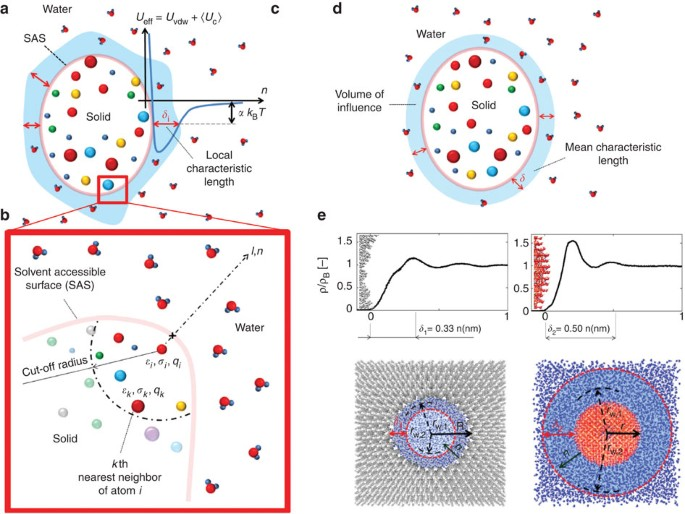Figure 2: The characteristic length of confinement δ.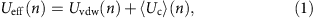along the n direction, orthogonally to the SAS and passing through the centre of the atom i (Fig. 2b). For the 12-6 Lennard–Jones potential, it follows that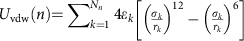, with εk, σk and rk denoting the depth of the potential well, the distance at with such potential becomes zero and the Euclidean distance between the generic line point with coordinate n and the centre of kth nearest neighbour, respectively. For the Coulomb interactions, the average potential energy at a fixed temperature T between the Nn atoms and the water dipoles is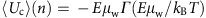, where E, μw, kB and Γ denote the electrical field strength, water dipole moment (7.50 × 10−30 C m for the SPC/E model), the Boltzmann constant and the Langevin function Γ(x)=coth(x)−1/x. The strength of the electrical field E can be readily computed following the law of electrostatics (see Methods). Knowing the effective potential Ueff(n) for the atom i, a corresponding characteristic length δi can be estimated within which the water molecules have reduced mobility. This length δi is given by δi=ni,2ni,1 where ni,2 and ni,1 are the two zeros of the equation Ueff(n)+kBT/4=0 (Fig. 2c). Therefore, based on the definition of δi, all the water molecules located within such a distance are significantly affected by the van der Walls and Coulomb interactions, whereas all the water molecules beyond the characteristic length δi can escape the potential well generated by the solid wall. Here kBT/4 is half the kinetic energy per independent degree of freedom according to the equipartition of energy (see Methods). By proper averaging over the surface, the mean characteristic length δ of the overall solid surface (Fig. 2d) can be derived as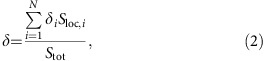with Sloc,i and N being the specific (per atom) SAS for the atom i and the total number of atoms, respectively. Note that the above formulation is general and applies to hydrophilic and hydrophobic surfaces, regardless of their electrostatic surface charge. Also, the characteristic length δ can be conveniently computed based on the geometry of the problem, Lennard–Jones force field parameters and the partial electrostatic charges using the script provided in the Supplementary Software 1.

In Fig. 2e, the water density profile within (a) a SiO2 nanopore and (b) around a single Fe3O4 NP is shown, where peaks denote the typical water layers nearby a solid wall at the nanoscale2,39. It is noteworthy that despite the great difference in the potential strength between SiO2 and Fe3O4 (min(Ueff,2)/min(Ueff,1)≈2.7), the corresponding difference in terms of characteristic lengths is much more moderate (δ2/δ1≈1.5). From the density profiles, Fe3O4 clearly induces a stronger perturbation in the nearby water molecules’ distribution. However, except for a first thin water layer strongly adsorbed to the NP surface (and accounted by δ2>δ1), the amplitude of these perturbations rapidly decays further away and becomes comparable in the remaining confined volume for both cases. Similarly, although there is a significant difference in the potential minimum between green fluorescence protein and (5,5) chirality CNT (min(Ueff,2)/min(Ueff,1)≈1.5), the difference between the two characteristic lengths is negligible. The above observations suggest that geometrical parameters could more significantly affect D as compared to energetic parameters.

Scaling law

Since water mobility is impaired mostly in a thin layer next to the liquid–solid interface with thickness δ, it is reasonable to assume that the observed variation in the self-diffusion coefficient D is mainly associated with the altered mobility of the water molecules within such a layer and the corresponding volume (volume of influence— Fig. 2d). In addition, it has been already observed that the self-diffusion coefficient D reduces as the ratio between the total interfacial area and the total volume occupied by water increases. On the basis of such an evidence, a scaling parameter θ can be introduced as the ratio between the total water volume of influence (Vin) and the total volume accessible to the water molecules (Vw); thus,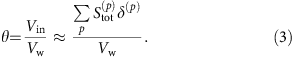This parameter θ varies from 0 (bulk water case) to 1 (totally confined water). The volume of influence (Vin) is the volume of water that feels the van der Walls and Coulomb interactions and is therefore influenced by the presence of solid walls. This volume is readily given by Vin≈∑pStot(p)δ(p), where Stot(p) and δ(p) represent the total SAS and characteristic length of the pth particle, respectively. As detailed in the Methods, possible overlap of the volumes of influence due to several particles/pores (for example, several NPs loaded within a SiO2 nanopore) can be easily taken into account by the continuum percolation theory (CPT), and a more accurate estimate of Vin may include the effect of particle curvature.

Assuming θ as the sole, independent variable for D, all computed values relax within a narrow band around a linear curve (Fig. 3) that can be readily described by the relationship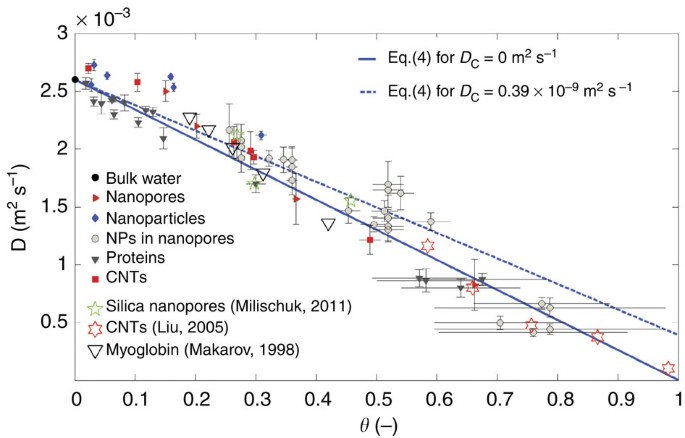Figure 3: Scaling behaviour of the water diffusion coefficient D.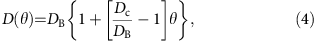where DB is the self-diffusion coefficient of bulk water, while DC the self-diffusion coefficient of totally confined water. Remarkably, Fig. 3 presents data from 58 different cases analysed in this work as well as data available in the published literature. Here despite the variety of the considered configurations, particles and sources of the results, a simple law is found to be sufficiently accurate to describe the phenomenon under study, thus confirming that θ is indeed an important controlling parameter under very diverse conditions and geometrical configurations. To have a more explicit formulation of equation (4), details about the evaluation of DC are provided in the section below.

Thermodynamic insights

Nanoconfined water shares some features with supercooled ordinary water in that it may not crystallize on cooling below the melting temperature of TM≈273.15 K (refs 1, 43). Within the thin δ layer of water molecules next to a solid surface, the thermodynamic state depends on the characteristic confinement length scale44. In particular, the specific heat capacity cp of nanoconfined water has been experimentally measured in narrow SiO2 nanopores and its variation with the temperature T is plotted in Fig. 4a for a pore diameter of 1.7 nm (ref. 45). Using these experimental data, the energy variation associated with the transition from bulk to confined water can be readily computed as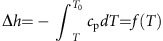, with f(T0)=0 at the bulk water temperature T0=300 K. From this, the inverse function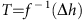can be derived as plotted in Fig. 4b. The energy variation associated with water nanoconfinement can also be written as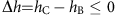, where hB and hC are the energy of bulk and confined water, respectively. Since the confined region is typically limited to 1–2 layers of water molecules (for all the considered structures, δ<0.6 nm), it can be assumed that h≈−ε, which is the minimum of the effective potential (Ueff) generated by the solid surface. The function f−1 can be used to compute the supercooled temperature of nanoconfined water corresponding to the energy variation −ε, that is, to say T=f−1(−ε). In the work by Chen et al.1, the diffusion coefficient of water molecules under strong nanoconfinement (that is, within SiO2 pores with diameter of 1.4–1.8 nm) is reported as a function of the temperature. From the latter experimental data, the diffusion coefficient of totally confined water DC can be readily expressed in the form DC=DB g(T) (Fig. 4c). Therefore, by combining the two sets of experimental data and knowing the value of ε, the diffusion coefficient of totally confined water DC can be computed as DC/DB=g′(T)=g′(f−1(−ε))=g(−ε). The ratio DC/DB is plotted in Fig. 4d for the different 58 cases analysed here. For the iron oxide NPs and CNTs, which are characterized by a fairly strong effective potential (large ε), DC≈0. As the strength of the effective potential reduces, the value for the diffusion coefficient of totally confined water increases. However, even for the SiO2 NPs and nanopores, and for the proteins that are characterized by lower ε, in our computations DC is at most 15% of DB. We stress that the function gh) is a property of water, and here we have chosen to estimate it on the basis of measurements in SiO2 nanopores only, because such experiments are among the very few that are well documented in literature.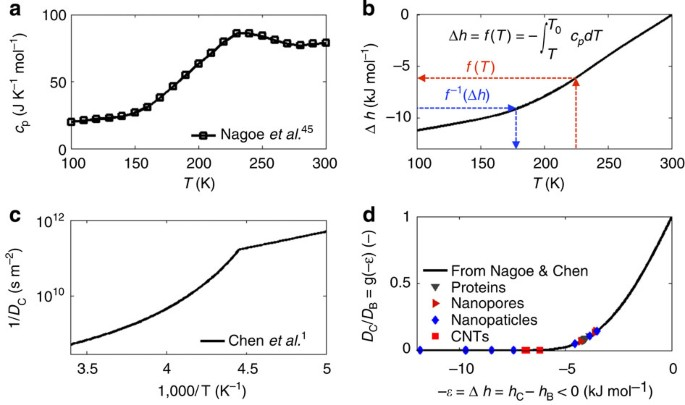Figure 4: Derivation of the function DC/DB=g(− ε).

In general, the volume of water can be partitioned into bulk (B) and confined (C). Invoking the mixing rule, the average diffusivity D of the system can be presented as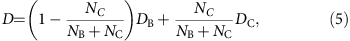where NC and NB are the number of water molecules in the confined and bulk regions, respectively. Considering that NC=ρCVC/mw, with ρC being the water (mean) density in the adsorbed region and mw being the mass of one water molecule, it follows: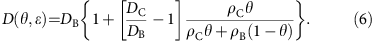This implies that the average diffusivity D depends in general on a geometrical parameter (θ) and an energetic parameter (ε). However, the following approximation (ρCθ)/(ρCθ+ρB(1−θ))≈θ can be safely made for the full range of θ (see Supplementary Fig. 4 and Supplementary Discussion). Therefore, equation (6) degenerates into equation (4) demonstrating that the diffusion of nanoconfined water can be interpreted invoking the thermodynamics of supercooled water. Moreover, based on the above discussion on DC (see Fig. 4d), for a large variety of nanoconfined systems, the simplifying assumption DC/DB≈0 can be safely made. In such cases, the much simpler law D(θ,ε)≈D(θ)=DB(1−θ) is readily derived showing the direct linear dependence of the water diffusion coefficient on the sole scaling parameter θ. Note that no empirical factor is needed to derive D(θ), with the latter law matching the values of the 58 MD simulations as well as 13 further configurations from the literature with a quite good coefficient of determination (R2=0.93, solid line in Fig. 3). The dashed line in Fig. 3 represents, instead, equation (4) with DC/DB=0.15, corresponding to the largest value of DC only observed in a few simulated cases (Fig. 4d).

Discussion

It has been shown that the relaxometric properties of contrast agents for MR imaging can be enhanced by proper modulation of the water molecule dynamics46. Recently, Decuzzi and collaborators23,24 have shown that the geometrical confinement of Gd3+-based MRI contrast agents and SPIOs within mesoporous structures can increase by several folds the longitudinal and transversal relaxivities, respectively, of the original agents. Here it is shown that the scaling law D(θ) can be effectively used to predict the enhancement in transversal relaxivity exhibited by SPIOs confined in mesoporous silicon structures. Referring to Fig. 5a, discoidal mesoporous silicon particles (SiMPs) of 1,000 nm in diameter and with an average pore size of 40 nm are synthesized by a combination of optical lithographic techniques and electrochemical etching26. These particles are exposed to a concentrated solution of SPIOs, which are loaded within the pores by capillary suction and form clusters of controlled size. As schematically shown in Fig. 5b and documented by the transmission electron microscopy (TEM) and energy-dispersive X-ray spectroscopy (EDX) images of Fig. 5c, the SPIOs are distributed more or less uniformly within the pores and across the porous matrix of the silicon particles. The black and red spots, respectively, in the TEM and EDX images identify the SPIO clusters within the mesoporous silicon matrix. In these experiments, commercially available SPIOs (Sigma-Aldrich) are considered, coated with a thin layer of polyethylene glycol to facilitate their dispersion in water, and presenting an average core diameter of 5.13±1.07 nm, as derived by TEM analysis. In bulk water the transversal relaxivity of the unconfined SPIOs has been measured to be (r2=107±24 mM−1 s−1), whereas on geometrical confinement within the SiMPs, the relaxivity rises up to r2=270±73 mM−1 s−1.

From the theory on the MR relaxation of iron oxide NPs, the transversal relaxivity r2 of the SPIOs dispersed in an aqueous solution can be estimated as29,32,47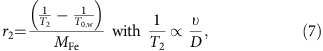where T2 and T0,w are the transversal relaxation times for the solution with contrast agents and bulk water (=2,800 ms), respectively; MFe is the iron concentration (mM) in the solution; υ is the volume fraction of the SPIOs; and D is the diffusion of the water molecules surrounding the SPIOs. Details on equation (7) are provided in the Supplementary Discussion.

For a fixed iron concentration MFe in the solution, the enhancement in transversal relaxivity is directly related to the ratio En=(υ/D)/(υB/DB), where the quantity υ/D has to be estimated for confined SPIOs and υB/DB for the free SPIOs dispersed in bulk water. Since the distribution of the SPIOs within the SiMP is not uniform, the diffusion coefficient D and the local SPIO volume fraction υ are expected to vary within the porous matrix. The local enhancement in relaxivity En is plotted in Fig. 5d. Areas are shown with mild and high enhancement, up to 20-fold, corresponding to different levels of SPIO loading and clustering, and therefore different levels of water confinement. By integrating over the whole silicon particle, an average relaxivity enhancement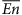can be computed as being ~2.7, which is in excellent agreement with the experimental value of ~2.52 (see Methods for details). Note that the proposed law can be used in several other fields to analyse different cases of water confinement.

In summary, it has been shown that the self-diffusion coefficient of nanoconfined water can be described by a unique dimensionless parameter θ, representing the ratio between the confined and total water volumes. The coefficient D scales linearly with θ and can be readily estimated, knowing the bulk DB and totally confined DC diffusion of water. This has been validated on the basis of almost 60 different cases and 5 different geometrical configurations, including the analysis of the water molecule dynamics within nanopores and CNTs, around NPs and proteins. The coefficient of diffusion for confined DC water is quantified on the basis of the thermodynamics of supercooled water. As an example, the scaling relation has been shown to accurately predict the enhancement in magnetic resonance relaxivity of iron oxide NPs confined into mesoporous silicon structures.

The proposed approach may be used to interpret experimental data collected in different scientific disciplines on the dynamics of water molecules under confined conditions and to rationally design nanostructures for modulating the diffusion of water. This is of relevance in nanomedicine, nanotechnology as well as in more traditional engineering fields such as heat transport, fluid dynamics and energy storage.

Methods

MD simulations

Atomic coordinates of Fe3O4 NPs are generated from Fe3O4 crystals48, whereas SiO2 crystals are considered in the case of nanopores and SiO2 NPs (Supplementary Figs 5–8)49. Crystal structures of proteins are taken from the Protein Data Bank ( http://www.rcsb.org; Supplementary Figs 9 and 10). CNTs are generated by means of the Visual Molecular Dynamics software (Supplementary Fig. 11)50. The SPC/E model51 is used for water molecules, which is known to accurately predict some of the properties of water relevant for this study at room temperature37. However, it is also worth noticing that the SPC/E model does not accurately predict some other properties of water. For instance, shear viscosity or thermal conductivity were found to be off by more than 50% at room temperature52. Bonded interactions of SiO2 and Fe3O4 are treated by means of harmonic stretching and angle potentials53. Van der Waals interactions are modelled by a 12-6 Lennard–Jones potential; partial charge interactions between solid surfaces and water are modelled by a Coulomb potential (Supplementary Tables 12–14)51,54,55,56. Non-zero partial charges are only assigned to atoms on the surface of nanopore or NP, which belong to silanol and FeOH groups, whereas all other atoms (bulk of SiO2 and Fe3O4) are considered as neutral.

Simulations are carried out with a leap-frog algorithm (time step: Δt=0.5 fs), and periodic boundary conditions are applied along the three Cartesian coordinates. After energy minimization of NP, nanopore or nanotube setups, the two subsystems (solid crystals and water) are initialized at 300 K (Maxwellian distribution of velocities) and fully coupled to a Nosé–Hoover thermostat57,58 (at 300 K and time constant τ=0.2 ps) for 50 ps, until the energies of the system relax to a steady state. During the latter preliminary calculation, one thermostat for each subsystem is adopted. Afterwards, Nosé–Hoover thermostats (at 300 K) are maintained attached to solid crystals only, whereas the simulation is continued up to 2 ns. In the case of proteins, the MD protocol is slightly changed to improve convergence. Energy minimization for proteins is performed before and after solvation and ions are added when needed for achieving the neutrality of the system, which is then equilibrated in two steps: 100 ps in canonical ensemble (fixed number N of particles, volume V and temperature T - NVT) at 300 K (initialization with Maxwellian distribution of velocities, Berendsen thermostats59 separately attached to proteins and water, τ=0.1 ps); 100 ps in NPT ensemble (fixed number N of particles, pressure P and temperature T) at 300 K and 1 bar (Berendsen thermostats separately attached to proteins and water, τ=0.1 ps; Parrinello–Rahman pressostat60 applied to the whole system, τ=2 ps). During the equilibration, all bonds in the proteins are kept rigid using the LINCS (Linear Constraint Solver) algorithm61. Finally, a Nosé–Hoover thermostat (300 K, τ=0.2 ps) is attached to protein’s atoms and the simulation is continued for 1 ns.

In all simulated cases, steady state is reached when D, which is evaluated every 200 ps, tends to an asymptotic value (Supplementary Figs 12–28 and Supplementary Note 2). This is generally achieved after about 600 ps, for all configurations. Note that the root mean square deviation of the proteins in the water box with respect to the crystallographic structures is on average found to be below 0.3 nm (Supplementary Fig. 29 and Supplementary Note 2). The self-diffusion coefficient D of the water molecules is determined following the classical relationship of Einstein and computing the mean square displacement as5,62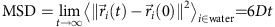, where the position vector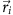refers to the centre of mass of the water molecule i at the generic time t and 0 refers to the initial configuration of the system. Alternative approaches for computing the water diffusivity could be considered as well (for example, those based on the first-passage concept)63,64. Further details on the implementation of the MD simulations and calculation of the self-diffusion coefficient D are provided in the Supplementary Methods.

The MD simulations are performed with the software package GROMACS65,66. Rendering is performed with UCSF Chimera67.

Derivation of the characteristic length δ

When defining the van der Waals potential Uvdw(n) in equation (1), the parameters εk and σk already incorporate a combination rule for the Lennard–Jones parameters between the atom i and oxygen atoms of water (for example, the Lorentz–Berthelot rule). Moreover, in the average potential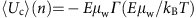, the effective strength of the electrical field E=|E(n)| may be expressed by the following explicit form: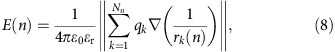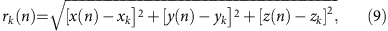with qk being the electric charge of the kth neighbour, while (x,y,z) and (xk,yk,zk) represent the Cartesian coordinates of the generic point on l (corresponding to the local coordinate n) and the Cartesian coordinates of neighbour k, respectively. In our approach, the relative permittivity εr is an input parameter to be provided to the Matlab(r) routine for the computation of δ (see below, Supplementary Fig. 30, Supplementary Methods and Supplementary Software 1). In particular, in this work, εr was included as a function of the distance from the particle εr(n) following the suggestion in ref. 68.

The expression of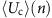is a classical result of electrostatics and can be justified as follows. Let E be the electric field acting on a dipole μ. The instantaneous energy can be expressed as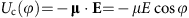, with E and μ being the field and dipole strength, respectively, while ϕ is the angle between the direction of the dipole and the field. The minimum and maximum values of energy are attained for ϕ=0 and π, respectively. However, in the presence of thermal agitation, the direction of μ (hence ϕ) is continuously changing in time. For classical systems, a number of independent dipoles are distributed according to their energy level Uc:Uc,min<Uc<Uc,max. In particular, at thermodynamic equilibrium, the Boltzmann distribution predicts that the number N(Uc) of dipoles with energy Uc is: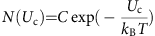, with C being a constant to be determined. Note that, in three dimensions, all dipoles lying on a cone with angle 2ϕ around the electrical field direction have the same energy. Moreover, at every ϕ, the total number of such dipoles is N(Uc(ϕ))dΩ, with Ω being the incremental solid angle. The above dipoles at ϕ give the component sum dμ in the field direction: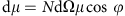. Hence, according to the Boltzmann distribution, the average dipole moment takes the more explicit form: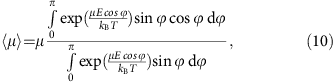On substituting β=μE/kBT and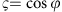, the above integral becomes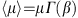.

On construction of the potential in equation (1) at an arbitrary atom i, a local characteristic length δi can be defined as δi=ni,2ni,1, where ni,2 and ni,1 are the two zeros of the equation (see Fig. 2c): Ueff(n)+αkBT=0, where we expect α≈1/4. In fact, provided that kBT/2 is the kinetic energy attributed the each degree of freedom of the water molecules, for planar surfaces α=1/4 because molecule are allowed to escape the potential well only along half of the direction orthogonal to the surface. The previous equation implies that water molecules located beyond the characteristic length δi are in the position of escaping the potential well generated by the Nn atoms in the solid wall owing to the kinetic energy kBT. Obviously, when the horizontal line U+αkBT=0 does not intersect the function in equation (1), δi=0.

In general, the quantity δi varies at each atom i (see Fig. 2a). Moreover, (meaningless) non-zero values for δi can be found for bulk atoms. Thus, for a given solid structure, it is convenient to define a mean characteristic length as in equation (2) (see Fig. 2d). Note that both Stot and Sloc,i are readily computed by GROMACS, once the geometry of the system is known (for example, in the form of a pdb file). It is also worth emphasizing that δ is a characteristic length of the whole system of interest and can be straightforwardly computed based on the geometry, Lennard–Jones force field parameters and partial charges.

The script for computing δ is based on Matlab(r) and it is provided as Supplementary Software 1 of this work.

Derivation of the scaling parameter θ

Finding a proper scaling parameter θ (or equivalently δ) is not trivial. A few unsuccessful attempts to find a general scaling parameter for water self-diffusion coefficient are reported in Supplementary Discussion and Supplementary Figs 31 and 32.

In equation (3) the particle curvature is neglected; thus, this is accurate in the limit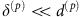, with d(p) being a representative radius of curvature of the pth particle. If the latter approximation is not properly fulfilled, a more accurate estimate of the numerator at the right-hand side of equation (3) can be easily adopted (see also a few examples in the Supplementary Discussion).

Clearly, in cases of strong confinement (and high values of θ) with the presence of several particles (for example, the reported studies where a number of spherical NPs are loaded within cylindrical nanopores), a partial overlap of the volumes of influence becomes more and more probable. However, we notice that volumes of influence due to different particles are not additive. As a result, the quantity θ computed by equation (3) is only apparent, with the effective fraction of the volume of influence being smaller than θ. The above issue is encountered in the framework of CPT. To this respect, a classical result of CPT, under the assumption of randomly placed volumes suggests that, to properly recover the effective fraction, the apparent volume fraction should be corrected as: 1−exp (−θ)69,70. Hence, we suggest that the above correction applies in the presence of overlap of the volumes of influence (for example, several particles within the computational box).

The volume Vw

For fully hydrated simple geometries, Vw can be computed by considering the nominal size of a particle/pore (see the discussion below about relaxivity enhancement). However, in general, the volume Vw in equation (3) can be defined as: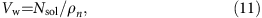where Nsol and ρn are the number of water molecules within the computational periodic box and the average water density, respectively. As a consequence, the volume occupied by a solvated particle p is Vp=VboxVw, where Vbox is the volume of the computational box. For complex configurations (for example, several NPs surrounded by water or within nanopores), the volume Vw can be estimated as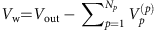, where Vp(p) and Np are the volume of the pth particle and the total number of particles, respectively, whereas Vout is the volume of the surrounding space (that is, Vout=Vbox for particles not loaded in nanopores, while Vout=Vpore for cases where particles are loaded within a pore whose volume, computed by equation (11), is Vpore).

Clearly, the use of equation (11) relies on the computation of the number density ρn. Using available packages in standard MD software, an estimate of the average value for ρn can be easily computed after solvation of a dry geometry. In our computations, we estimated the water volume in a few realizations of each configuration of interest, several measures of ρn were collected and used to compute an average value and the corresponding s.d. The latter s.d. generates uncertainties when computing the volume Vw, and consequently uncertainties of the scaling parameter (horizontal error bars in Fig. 3). Alternative approaches based, for instance, on Monte–Carlo integration can also be used to calculate Vw accurately.

Characterization of nanoconstructs and NPs

Details on the fabrication and characterization of the discoidal SiMPs and the SPIOs are provided in the Supplementary Methods and Supplementary Figs 33 and 34, together with the protocols for the loading of SPIOs into SiMPs.

Relaxivity measurements

In vitro relaxation times were measured in a Bruker Minispec (mq 60) bench-top relaxometer operating at 60 MHz and 37 °C. The transverse (T2) relaxation times were measured using the Carr–Purcell–Meiboom–Gill sequence.

Analysis of relaxivity enhancement

The function fEDX(X,Y) of the two spatial coordinates X and Y is built from the EDX image for iron (Fig. 5c) and denotes the position of the centre of a generic image pixel. Let NSPIO be the average number of SPIOs within a single SiMP. Hence, a surface density function at a pixel scale for the SPIOs can be written as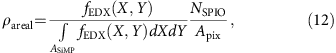where Apix and ASiMP are the surface area of a single pixel and the surface area of a SiMP, respectively. The function ρareal has been reported in the (left) bottom part of Supplementary Fig. 35. SiMP pores that are loaded by SPIOs can be automatically recognized by finding the local density map of the EDX non-zero elements (ρpoint). The density function in equation (12) depends on the image pixel size (that is, a quantity that is not representative of the phenomenon under study), whereas a more meaningful function should be based on the pore characteristic dimension instead (representative of the confinement length). Hence, the following surface density function at a pore scale for the SPIOs is introduced: ρSPIO(X,Y)=point(X,Y), with C being a constant to be determined. To this end, owing to mass conservation, it is imposed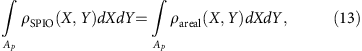hence,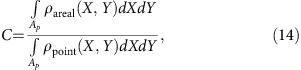with Ap being the surface area of a representative SiMP pore (see also the (right) bottom part of Supplementary Fig. 35). The total number of SPIOs within the representative pore can be computed as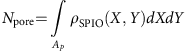. Hence, the water volume near the SPIOs (for the latter pore) Vw can be estimated as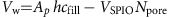, where h is the SiMP height, while 0≤ cfill≤1 indicates the fraction of the SiMP pore that has been effectively occupied by SPIOs. Clearly, for homogeneously distributed SPIOs within the pore, cfill=1. For the considered representative pore, an estimate of the average scaling parameter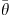can be obtained as: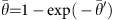with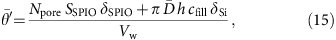where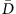is the average diameter of the pores. Finally, a map of the scaling parameter θ=θ(X,Y) is derived by the map ρSPIO as θ(X,Y)=CρSPIO(X,Y), where the constant C′ is determined by imposing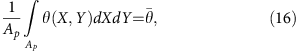hence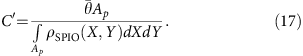On the estimate of the scaling parameter map θ=θ(X,Y), the computation of a corresponding map for the diffusion coefficient D=D(X,Y) is straightforwardly achieved by adopting the law suggested in this work.

The above procedure is slightly sensitive to the choice of the representative pore. However, from our computations no significant changes in the final result (map of θ=θ(X,Y)) was experienced by making difference choices. In Supplementary Fig. 36, we report both the map of the scaling parameter θ=θ(X,Y) and the corresponding D=D(X,Y) at different pore filling.

Let us consider two contrast agents with the same iron molarity MFe. Let the first one be based on SPIOs homogenously dispersed in bulk water, while the second one adopts the same SPIOs (nanoconfined) within SiMPs. Given the definition of r2 (Supplementary equation (9)), we can define a relaxivity enhancement as follows: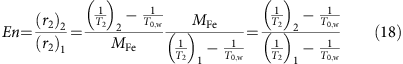Since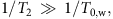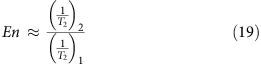Finally, using the outer sphere theory (see Supplementary Discussion), the enhancement can be recast as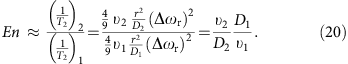Consistently with the above procedure, we aim at estimating the map En(X,Y):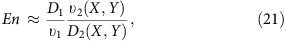where D1 and υ1 are the self-diffusion coefficient of bulk water and the average volume fraction of SPIOs, respectively. Let us assume that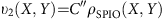with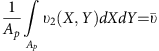; hence,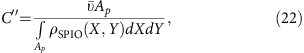and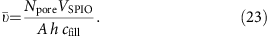In Fig. 5d we report the function En(X,Y) corresponding to the SiMP particle of Fig. 5c.

Finally, the average enhancement due to the entire SiMP can be estimated as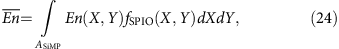where fSPIO(X,Y) is the following distribution function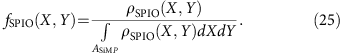For the case in Fig. 5d, we find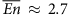. In Fig. 5c, we consider SiMPs filled by 5 nm SPIOs, the transverse relaxivity of which was experimentally assessed as (r2)2=270±73 mM−1 s−1. Moreover, 5 nm SPIOs (Sigma-Aldrich) show transverse relaxivity in bulk conditions of water (r2)1=107±24 mM−1 s−1. As a result, the experimentally measured enhancement for the agent in Fig. 5c (compared with SPIOs in bulk water) is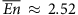, which is in excellent agreement with the above prediction, fully based on the EDX signal (iron) and our model.

How to cite this article: Chiavazzo, E. et al. Scaling behaviour for the water transport in nanoconfined geometries. Nat. Commun. 5:3565 doi: 10.1038/ncomms4565 (2014).

References

1. 1

Chen, S.-H. et al. The violation of the Stokes–Einstein relation in supercooled water. Proc. Natl Acad. Sci. USA 103, 12974–12978 (2006).

2. 2

Majolino, D., Corsaro, C., Crupi, V., Venuti, V. & Wanderlingh, U. Water diffusion in nanoporous glass: an NMR study at different hydration levels. J. Phys. Chem. B 112, 3927–3930 (2008).

3. 3

Nguyen, T. X. & Bhatia, S. K. Some anomalies in the self-diffusion of water in disordered carbons. J. Phys. Chem. C 116, 3667–3676 (2012).

4. 4

Sendner, C., Horinek, D., Bocquet, L. & Netz, R. R. Interfacial water at hydrophobic and hydrophilic surfaces: Slip, viscosity, and diffusion. Langmuir 25, 10768–10781 (2009).

5. 5

Allen, M. P. & Tildesley, D. J. Computer Simulation of Liquids Oxford Univ. Press (1989).

6. 6

Pérez-Hernández, N. et al. The mobility of water molecules through hydrated pores. J. Phys. Chem. C 116, 9616–9630 (2012).

7. 7

Zang, J., Konduri, S., Nair, S. & Sholl, D. S. Self-diffusion of water and simple alcohols in single-walled aluminosilicate nanotubes. ACS Nano 3, 1548–1556 (2009).

8. 8

Carrasco, J., Hodgson, A. & Michaelides, A. A molecular perspective of water at metal interfaces. Nat. Mater. 11, 667–674 (2012).

9. 9

N’Tsoukpoe, K. E., Liu, H., Le Pierrès, N. & Luo, L. A review on long-term sorption solar energy storage. Renew. Sust. Energ. Rev. 13, 2385–2396 (2009).

10. 10

Yu, N., Wang, R. Z. & Wang, L. W. Sorption thermal storage for solar energy. Progr. Energ. Combust. Sci. 39, 489–514 (2013).

11. 11

Cohen-Tanugi, D. & Grossman, J. C. Water desalination across nanoporous graphene. Nano Lett. 12, 3602–3608 (2012).

12. 12

Schmidt-Rohr, K. & Chen, Q. Parallel cylindrical water nanochannels in Nafion fuel-cell membranes. Nat. Mater. 7, 75–83 (2007).

13. 13

Hu, M., Goicochea, J. V., Michel, B. & Poulikakos, D. Water nanoconfinement induced thermal enhancement at hydrophilic quartz interfaces. Nano Lett. 10, 279–285 (2009).

14. 14

Murshed, S., Leong, K. & Yang, C. Thermophysical and electrokinetic properties of nanofluids–a critical review. Appl. Therm. Eng. 28, 2109–2125 (2008).

15. 15

Gates, B. D. et al. New approaches to nanofabrication: molding, printing, and other techniques. Chem. Rev. 105, 1171–1196 (2005).

16. 16

Wang, J., Zheng, Z., Li, H., Huck, W. & Sirringhaus, H. Dewetting of conducting polymer inkjet droplets on patterned surfaces. Nat. Mater. 3, 171–176 (2004).

17. 17

Tait, M. J., Saadoun, S., Bell, B. A. & Papadopoulos, M. C. Water movements in the brain: role of aquaporins. Trends Neurosci. 31, 37–43 (2008).

18. 18

Fechete, R., Demco, D., Eliav, U., Blümich, B. & Navon, G. Self‐diffusion anisotropy of water in sheep Achilles tendon. NMR Biomed. 18, 577–586 (2005).

19. 19

Paran, Y., Bendel, P., Margalit, R. & Degani, H. Water diffusion in the different microenvironments of breast cancer. NMR Biomed. 17, 170–180 (2004).

20. 20

Smouha, E. & Neeman, M. Compartmentation of intracellular water in multicellular tumor spheroids: diffusion and relaxation NMR. Magn. Reson. Med. 46, 68–77 (2001).

21. 21

Barron, L. D., Hecht, L. & Wilson, G. The lubricant of life: a proposal that solvent water promotes extremely fast conformational fluctuations in mobile heteropolypeptide structure. Biochemistry 36, 13143–13147 (1997).

22. 22

Zhou, H.-X., Rivas, G. & Minton, A. P. Macromolecular crowding and confinement: biochemical, biophysical, and potential physiological consequences. Annu. Rev. Biophys. 37, 375 (2008).

23. 23

Ananta, J. S. et al. Geometrical confinement of gadolinium-based contrast agents in nanoporous particles enhances T1 contrast. Nat. Nanotech. 5, 815–821 (2010).

24. 24

Sethi, R. et al. Enhanced MRI relaxivity of Gd3+‐based contrast agents geometrically confined within porous nanoconstructs. Contrast Media Mol. Imaging 7, 501–508 (2012).

25. 25

Liu, Z. et al. Drug delivery with carbon nanotubes for in vivo cancer treatment. Cancer Res. 68, 6652–6660 (2008).

26. 26

Tasciotti, E. et al. Mesoporous silicon particles as a multistage delivery system for imaging and therapeutic applications. Nat. Nanotech. 3, 151–157 (2008).

27. 27

Key, J. et al. Engineering discoidal polymeric nanoconstructs with enhanced magneto-optical properties for tumor imaging. Biomaterials 34, 5402–5410 (2013).

28. 28

Wu, Y., Joseph, S. & Aluru, N. Effect of cross-linking on the diffusion of water, ions, and small molecules in hydrogels. J. Phys. Chem. B 113, 3512–3520 (2009).

29. 29

Brooks, R. A., Moiny, F. & Gillis, P. On T2‐shortening by weakly magnetized particles: the chemical exchange model. Magn. Reson. Med. 45, 1014–1020 (2001).

30. 30

Caravan, P. Strategies for increasing the sensitivity of gadolinium based MRI contrast agents. Chem. Soc. Rev. 35, 512–523 (2006).

31. 31

Lauffer, R. B. Paramagnetic metal complexes as water proton relaxation agents for NMR imaging: theory and design. Chem. Rev. 87, 901–927 (1987).

32. 32

Tong, S., Hou, S., Zheng, Z., Zhou, J. & Bao, G. Coating optimization of superparamagnetic iron oxide nanoparticles for high T2 relaxivity. Nano Lett. 10, 4607–4613 (2010).

33. 33

Chiavazzo, E. & Asinari, P. Enhancing surface heat transfer by carbon nanofins: towards an alternative to nanofluids? Nanoscale Res. Lett. 6, 1–13 (2011).

34. 34

Schuler, L. D., Daura, X. & van Gunsteren, W. F. An improved GROMOS96 force field for aliphatic hydrocarbons in the condensed phase. J. Comput. Chem. 22, 1205–1218 (2001).

35. 35

Lervik, A., Bresme, F., Kjelstrup, S., Bedeaux, D. & Miguel Rubi, J. Heat transfer in protein-water interfaces. Phys. Chem. Chem. Phys. 12, 1610–1617 (2010).

36. 36

Mu, Y., Kosov, D. S. & Stock, G. Conformational dynamics of trialanine in water. 2. Comparison of AMBER, CHARMM, GROMOS, and OPLS Force Fields to NMR and infrared experiments. J. Phys. Chem. B 107, 5064–5073 (2003).

37. 37

van der Spoel, D., van Maaren, P. J. & Berendsen, H. J. A systematic study of water models for molecular simulation: derivation of water models optimized for use with a reaction field. J. Chem. Phys. 108, 10220 (1998).

38. 38

Asay, D. B. & Kim, S. H. Evolution of the adsorbed water layer structure on silicon oxide at room temperature. J. Phys. Chem. B 109, 16760–16763 (2005).

39. 39

Gallo, P., Rovere, M. & Spohr, E. Supercooled confined water and the mode coupling crossover temperature. Phys. Rev. Lett. 85, 4317–4320 (2000).

40. 40

Eisenhaber, F., Lijnzaad, P., Argos, P., Sander, C. & Scharf, M. The double cubic lattice method: efficient approaches to numerical integration of surface area and volume and to dot surface contouring of molecular assemblies. J. Comput. Chem. 16, 273–284 (1995).

41. 41

Lee, B. & Richards, F. M. The interpretation of protein structures: estimation of static accessibility. J. Mol. Biol. 55, 379–400 (1971).

42. 42

Shrake, A. & Rupley, J. A. Environment and exposure to solvent of protein atoms. Lysozyme and insulin. J. Mol. Biol. 79, 351–371 (1973).

43. 43

Poole, P. H., Sciortino, F., Essmann, U. & Stanley, H. E. Phase behaviour of metastable water. Nature 360, 324–328 (1992).

44. 44

Swenson, J., Jansson, H. & Bergman, R. Relaxation processes in supercooled confined water and implications for protein dynamics. Phys. Rev. Lett. 96, 247802 (2006).

45. 45

Nagoe, A., Kanke, Y., Oguni, M. & Namba, S. Findings of Cp maximum at 233 K for the water within silica nanopores and very weak dependence of the tmax on the pore size. J. Phys. Chem. B 114, 13940–13943 (2010).

46. 46

Laurent, S. et al. Magnetic iron oxide nanoparticles: synthesis, stabilization, vectorization, physicochemical characterizations, and biological applications. Chem. Rev. 108, 2064–2110 (2008).

47. 47

Gillis, P., Moiny, F. & Brooks, R. A. On T2‐shortening by strongly magnetized spheres: a partial refocusing model. Magn. Reson. Med. 47, 257–263 (2002).

48. 48

Okudera, H., Kihara, K. & Matsumoto, T. Temperature dependence of structure parameters in natural magnetite: single crystal X-ray studies from 126 to 773 K. Acta Crystallogr. Sect. B Struct. Sci. 52, 450–457 (1996).

49. 49

Kihara, K. An X-ray study of the temperature dependence of the quartz structure. Eur. J. Mineral 2, 63–77 (1990).

50. 50

Humphrey, W., Dalke, A. & Schulten, K. VMD: visual molecular dynamics. J. Mol. Graphics 14, 33–38 (1996).

51. 51

Berendsen, H., Grigera, J. & Straatsma, T. The missing term in effective pair potentials. J. Phys. Chem. 91, 6269–6271 (1987).

52. 52

Mao, Y. & Zhang, Y. Thermal conductivity, shear viscosity and specific heat of rigid water models. Chem. Phys. Lett. 542, 37–41 (2012).

53. 53

Lopes, P. E., Murashov, V., Tazi, M., Demchuk, E. & MacKerell, A. D. Development of an empirical force field for silica. Application to the quartz-water interface. J. Phys. Chem. B 110, 2782–2792 (2006).

54. 54

Lümmen, N. & Kraska, T. Investigation of the formation of iron nanoparticles from the gas phase by molecular dynamics simulation. Nanotechnology 15, 525 (2004).

55. 55

Malani, A., Ayappa, K. & Murad, S. Influence of hydrophilic surface specificity on the structural properties of confined water. J. Phys. Chem. B 113, 13825–13839 (2009).

56. 56

Soontrapa, C. & Chen, Y. Optimization approach in variable-charge potential for metal/metal oxide systems. Comput. Mater. Sci. 46, 887–892 (2009).

57. 57

Hoover, W. G. Canonical dynamics: equilibrium phase-space distributions. Phys. Rev. A 31, 1695 (1985).

58. 58

Nosé, S. A unified formulation of the constant temperature molecular dynamics methods. J. Chem. Phys. 81, 511 (1984).

59. 59

Berendsen, H. J., Postma, J. P. M., van Gunsteren, W. F., DiNola, A. & Haak, J. Molecular dynamics with coupling to an external bath. J. Chem. Phys. 81, 3684 (1984).

60. 60

Nosé, S. & Klein, M. L. Constant pressure molecular dynamics for molecular systems. Mol. Phys. 50, 1055–1076 (1983).

61. 61

Hess, B., Bekker, H., Berendsen, H. J. & Fraaije, J. G. LINCS: a linear constraint solver for molecular simulations. J. Comput. Chem. 18, 1463–1472 (1997).

62. 62

Mark, P. & Nilsson, L. Structure and dynamics of the TIP3P, SPC, and SPC/E water models at 298 K. J. Phys. Chem. A 105, 9954–9960 (2001).

63. 63

Malgaretti, P., Pagonabarraga, I. & Rubi, M. Entropic transport in confined media: a challenge for computational studies in biological and soft-matter systems. Front. Phys. 1, 21 (2013).

64. 64

Redner, S. A Guide to First-Passage Processes Cambridge Univ. Press (2001).

65. 65

Hess, B., Kutzner, C., van der Spoel, D. & Lindahl, E. GROMACS 4: algorithms for highly efficient, load-balanced, and scalable molecular simulation. J. Chem. Theory Comput. 4, 435–447 (2008).

66. 66

Lindahl, E., Hess, B. & Van Der Spoel, D. GROMACS 3.0: a package for molecular simulation and trajectory analysis. J. Mol. Model. 7, 306–317 (2001).

67. 67

Pettersen, E. F. et al. UCSF Chimera—a visualization system for exploratory research and analysis. J. Comput. Chem. 25, 1605–1612 (2004).

68. 68

Gongadze, E. et al. Ions and water molecules in an electrolyte solution in contact with charged and dipolar surfaces. Electrochim. Acta. (2013) (In Press).

69. 69

Stauffer, D. & Aharony, A. Introduction to Percolation Theory CRC Press (1994).

70. 70

Chiavazzo, E. & Asinari, P. Reconstruction and modeling of 3D percolation networks of carbon fillers in a polymer matrix. Int. J. Therm. Sci. 49, 2272–2281 (2010).

Acknowledgements

This work was supported by the Cancer Prevention Research Institute of Texas through the Grant CPRIT RP110262. This work was also partially supported through grants from the National Institutes of Health (USA) (NIH) U54CA143837 and U54CA151668. P.A. and E.C. acknowledge the support of the Italian Ministry of Research (FIRB Grant RBFR10VZUG). E.C. is grateful to the US-Italy Fulbright Commission for partial support. M.F. acknowledges travel support from the Scuola Interpolitecnica di Dottorato—SCUDO. We thank the reviewers for useful comments and suggestions.

Author information

E.C. and M.F. designed the computational plans. M.F. performed all the MD simulations. P.A. and E.C. conceived the idea of the suggested scaling. P.D. conceived the idea of the study, and with P.A. designed and coordinated the project. All the authors interpreted and discussed the computational and experimental results and wrote the manuscript.

Correspondence to Pietro Asinari.

Ethics declarations

Competing interests

The authors declare no competing financial interests.

Supplementary information

Supplementary Figures, Tables, Notes, Discussion, Methods and References

Supplementary Figures 1-36, Supplementary Tables 1-14, Supplementary Notes 1-2, Supplementary Discussion, Supplementary Methods and Supplementary References (PDF 4145 kb)

Supplementary Software 1

Matlab(r) routine for computing characteristic length of the confinement (ZIP 7 kb)

Rights and permissions

Reprints and Permissions

• Structure-Relaxivity Relationships of Magnetic Nanoparticles for Magnetic Resonance Imaging

• Zijian Zhou
• , Lijiao Yang
• , Jinhao Gao
•  & Xiaoyuan Chen

• From GROMACS to LAMMPS: GRO2LAM

• Hernán Chávez Thielemann
• , Annalisa Cardellini
• , Matteo Fasano
• , Luca Bergamasco
• , Matteo Alberghini
• , Gianmarco Ciorra
• , Eliodoro Chiavazzo
•  & Pietro Asinari

Journal of Molecular Modeling (2019)

• Fluidity and phase transitions of water in hydrophobic and hydrophilic nanotubes

• Mohamed Shaat
•  & Yongmei Zheng

Scientific Reports (2019)

• Protein Solvent Shell Structure Provides Rapid Analysis of Hydration Dynamics

• Jayangika N. Dahanayake
• , Elaheh Shahryari
• , Kirsten M. Roberts
• , Micah E. Heikes
• , Chandana Kasireddy
•  & Katie R. Mitchell-Koch

Journal of Chemical Information and Modeling (2019)

• ENHANCED OIL FLOW MODEL COUPLING FRACTAL ROUGHNESS AND HETEROGENEOUS WETTABILITY

• HAIMING FAN
• , HUI LI
•  & HAN WANG

Fractals (2019)